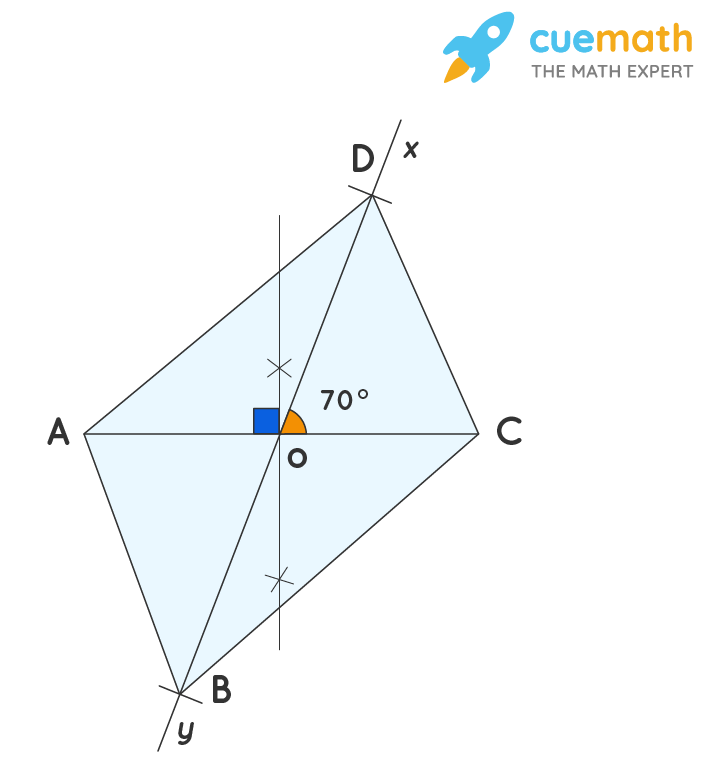# Construct a Parallelogram Whose Diagonal Is 5.4cm and 6.2 and an Angle Between Them Is 70.

## Question: Construct a parallelogram whose diagonal is 5.4cm and 6.2 and an angle between them is 70.

A parallelogram is a type of quadrilateral in which the opposite sides are parallel and equal.

## Answer: The construction of the parallelogram is discussed below.

Let's follow the step of construction.

## Explanation:

In a parallelogram, diagonals bisect each other.The following are the steps of construction to draw a parallelogram ABCD with the given parameters:

• Draw AC = 5.4 cm.
• Construct perpendicular bisector of AC i.e., Bisect AC at O.
• Draw ∠COX = 70° and produce XO to Y.
• OB = 1/2 diagonal BD = 1/2 (6.2) = 3.1 cm and OD = 1/2 (6.2) =3.1 cm as shown.
• Join AB, BC, CD, and DA.